Tuesday, March 07, 2006

Verbogeometry -- Installment VII --- Pythagorean Theorem and The Distance Formula

2.6. The Distance Formula and Pythagorean Theorem: The distance formula uses the Pythagorean Theorem to calculate distances on the Cartesian coordinate system. The Pythagorean Theorem says that the hypotenuse of a right triangle is equal to the square of it sides. (see figure 26)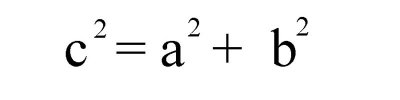Figure. 26

solve for c and we get the following see figure 27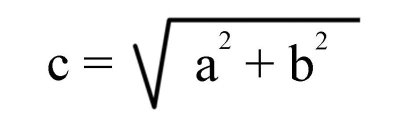Figure. 27

Let us plot two points on a two-dimensional axis system P1(-9,10) and P2(4,3) and If we draw a lines between the points and lines parallel to the axes we can obtain a right triangle. (see figure 28)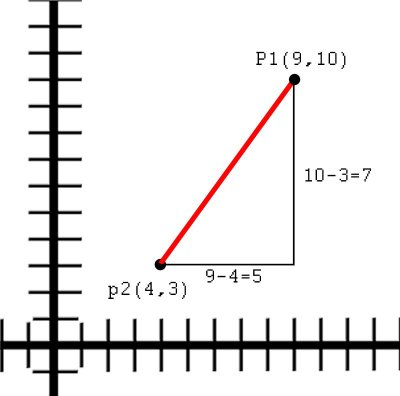Figure. 28

To solve the length of the hypotenuse we first find the difference between the x values and the y values to create the sides of the triangle then we plug the values into the Pythagorean Theorem.
x value is 9 - 4 = 5 and the y value is 10 - 3 = 7
Now we plug it to the equation and we get the expression in figure 29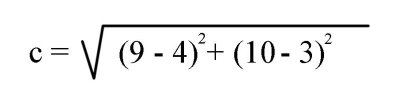Figure 29

The distance or length of the hypotenuse would be the square root of 74 or approximately 8.602
The distance formula is thus:
Given two points (x1,y1) and (x2,y2) (see figure 30)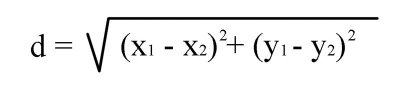Figure 30

Now what we have been looking at up till now has been in two-dimensions but we can also express a distance in many dimensions. Let us look at two points in three dimensions.
P1(x1,y1,z1) P2(x2,y2,z2)
If we were to put these two points into the distance formula it would look like what is shown in figure 31Figure 31

It is evident that we can use the distance formula in uncountable dimensions all we have to is to add another dimensional term to the formula for every dimension we wish to express.

Monday, March 06, 2006

Verbogeometry -- Installment VI --- Prismatic Structures

2.5. Prismatic Structures in Verbogeometry: Notice that 'barren and infertile' is a complex antonym and 'fertile and infertile' is a simple antonym as previously defined. This is interesting because we can see that there exists in verbogeometry a geometric construction where a line expressed as a simple antonym is normal (90 degrees) to a plane containing all of the complex antonyms related to the line which is expressing the simple antonym. To illustrate this idea lets look again at the relationship between the simple antonyms 'fertile' and 'infertile' and the synonyms 'barren', 'fruitless', 'unproductive', 'sterile', 'impotent' which reside on the plane that is normal (90 degrees) to the line created by the simple antonyms. Furthermore you can draw lines from all of the synonyms back to the complex antonym 'fertile'. (See figure 19)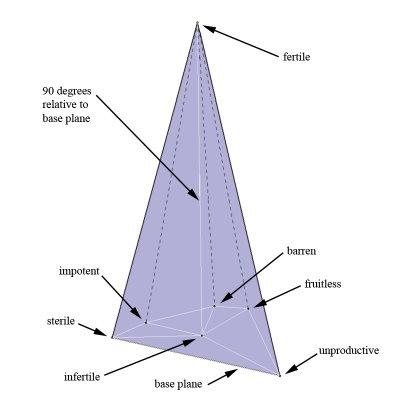Figure 19.

This idea also lends itself to prismatic structures where we have a group of parallel simple antonyms whose endpoints construct polygonal faces on two parallel synonym-planes. (See figure 19) Example: Let us define one synonym plane containing the words 'pleased', 'content', 'affected', 'satisfied', 'enchanted' and 'sympathetic'. The other plane contains the following simple antonyms for the previous group of synonyms: 'displeased', 'discontent', 'disaffected', 'dissatisfied', 'disenchanted' and 'unsympathetic'. Due to the synonyms of one plane have corresponding simple antonyms which create lines 90 degrees from the synonym-plane then the simple antonyms are synonyms of each other and reside on their own individual synonym plane and because the lines are 90 degrees to each other the planes must be parallel. The former verbiage is a lot easier to understand visually (See figure 20)Figure 20.

On a side note: Everything that we have been talking about up to this point has been viewed in Euclidian space. Not much time has been spent exploring verbogeometry outside of Euclidian space. However, I see that there can be a lot more thought evolving models of verbogeometry in other spaces. For example, Instead of viewing antonyms and all the varying meanings spread out across a number-line, we may think of the antonyms as magnetic dipoles. Furthermore, all the varying meaning between them is analogous to a magnetic flux. Yet again, another view may be looking at the antonyms residing at the endpoints of a major axis of a three dimensional ellipsoid and the varying meanings reside on the surface of the ellipsoid. (See figure 21)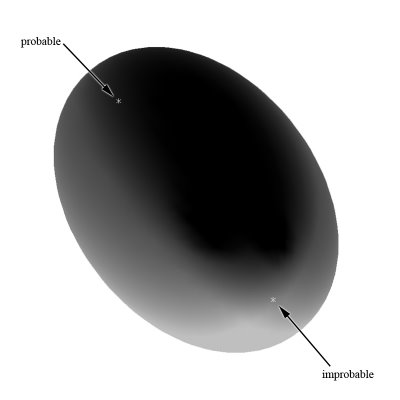Figure 21.

Figure 22 shows an ellipsoidal flux-like paradigm for the previous prismatic structure. (See figure 20)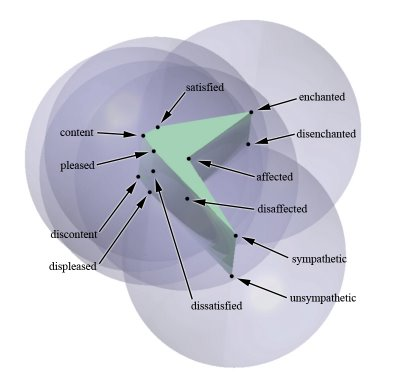Figure 22.

Figure23 shows an unlabeled side viewFigure 23.

It may be easier to see the ellipse structure if it was wireframe (See figure 24)Figure 24.

Figure 25 shows 5 parallel word axes in a wireframe structureFigure 25.

Sunday, March 05, 2006

Verbogeometry -- Installment V --- With Trigonometry

2.4. Verbogeometry with Trigonometry: First let us solve a pertinent traditional trigonometric problem, which requires a solution for an angle. Let us make 'theta' the variable of the angle in question. The tangent of a right triangle is defined as the ratio of the side opposite the angle 'theta' to the side adjacent to the angle 'theta'. If we call the side opposite distance "y" and the side adjacent as distance "x" we then arrive at tan (angle theta) = y/x (See figure 16)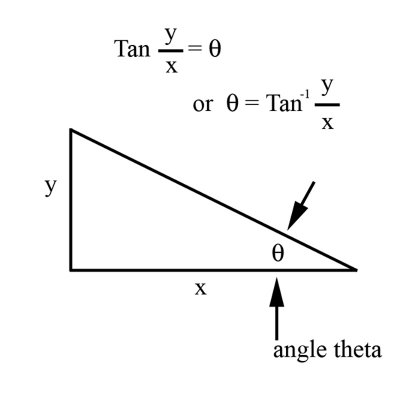Figure 16.

It also follows that if we know the distance of two sides of a right triangle we can find all the angles. Let us look at an example with the side opposite angle theta being 3 and the side adjacent to angle theta being 4 and. Let us now solve for the angle theta. We know that y/x = tan (theta) so it follows ¾ = tan (theta). To uncover the value of theta, we must take the inverse tangent of ¾. Symbolically this is stated as "theta = inverse tan(y/x)" or approximately 36.87 degrees (See figure 17).Figure 17.

In general, to reveal the angle after you have calculated the value for the tangent of y/x, your choice is to find the angle on a trigonometric table (old method) or you plug the values in on a hand held calculator.
Now let us look at a similar expression using verbogeometry and looking for the value of angle theta. This time we define the y distance as the difference between the concepts of barren and infertile (think number-line again) and let us define the x distance as the difference between the concepts of infertile and fertile. (See figure 18)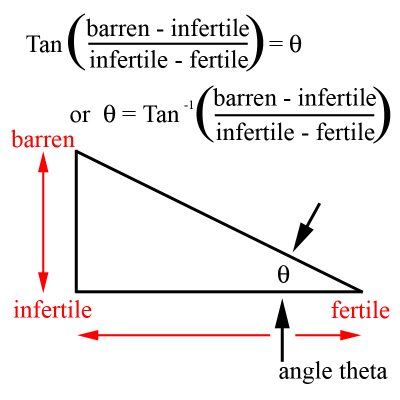Figure 18.

If we want to know the angle of theta we have to take the inverse tangent of y/x or the inverse tangent of (barren - infertile)/(fertile - infertile) (see figure 18)

Saturday, March 04, 2006

Verbogeometry -- installment IV --- Midpoint Formula

2.3. Midpoint Formula in Verbogeometry: Any analytic geometry equation can use coordinate word-pairs instead of numbers to express poetic forms. Let us use the midpoint formula to express the exact point between the two points P1(praise,love) and P2(punishment,hate) from figure 13. Before we look at coordinate word-pairs let us refresh the use of the midpoint formula in analytic geometry. To find the midpoint between two points on a Cartesian coordinate system we add the x coordinates together and divide by 2 to find the x value for the midpoint and we also add the y coordinates together and divide by 2 to find the y value for the midpoint or
(x1 + x2)/2 = x0 and (y1 + y2)/2 = y0.
Lets look at an example of finding the midpoint P0 between the points P1(-14-15) and P2(12,11) utilizing the midpoint formula. x1 = -14 and x2 = 12 so substituting our numbers in the variables of the equation (x1 + x2)/2 = x0 we get (-14 + 12)/2 = -1 also y1 = -15 and y2 = 11 so substituting our numbers in the variables of the equation (y1 + y2)/2 = y0 we get (-15 + 11)/2 = -2 therefore: P0 = (-1,-2) (see figure 14.)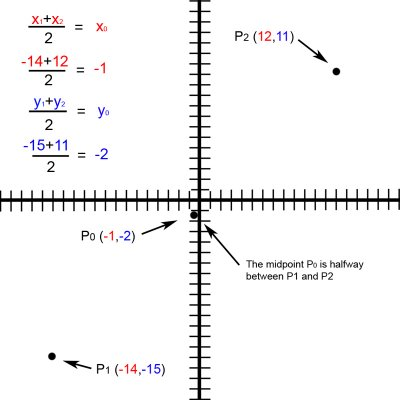Figure 14.

Let us now take a different approach and replace the numeric variables in the midpoint equation with the words/concepts of love, hate, praise and punishment. We will use the form of coordinate word-pairs P2(love, praise) and P1(hate, punishment). The midpoint formula now shows us that P0(x0, y0) will be formed by the substitution of (x1 + x2)/2 = x0 with (love + hate) = x0 and (y1 + y2)/2 = y0 with (hate + punishment) = y0. Now we have expressed the exact point between love, praise and hate, punishment. (see figure 15.)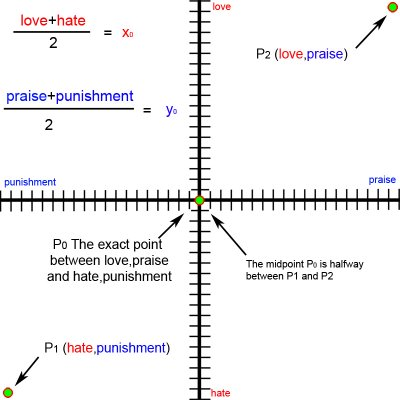Here is a relative piece from 2000 http://www.kazmaslanka.com/midpoint.html

Friday, March 03, 2006

Verbogeometry -- installment III --- Word-coordinate Pairs

2.2. Word-coordinate Pairs: We have witnessed a word-axis with different values of an antonym pair along a particular axis 'x' or 'y' in one dimension. (See figure 3) We also have seen a word-plane with values of two antonym pairs along two axes 'x' and 'y' in two dimensions. (See figure 10) Furthermore, we can have word-cubes along the x, y, and z-axes in the third and word-hypercubes in the fourth dimension or we can have antonymic pairs in innumerable dimensions. There is no limit to the dimensional palette for our expressions. Each antonym word-pair adds a new spatial dimension to our expressive construction. Let us talk about the spatial accuracy in defining the location of words in space. Once again, let us look at figure 10 and notice the antonym word pairs, just/unjust and noble/ignoble. However, let us focus our attention to the word-axis just/unjust. We know that we have defined a one-dimensional word-axis with different values of just and unjust but we do not know exactly where each of the words is located along the axis. We have no quantitative value for just or unjust. However, we do have a qualitative value and we know that the word exists somewhere on the axis. What is most important to us in verbogeometry is not the value as such, but the spatial relationship of the values to each other in space. Because the value or the meaning of a word is relative to the context in which it is used, each viewer individually creates his or her own context for meaning. Therefore, exact quantification of the word or its location in space is not possible. However, in some cases, it may be possible to restrict the context to a level where repeatable correlations exist, but those studies are more akin to denotation for the purpose of science. Scientific experimentation "proves" the equation to be mathematically correct and workable within a range of acceptability. In other words, experimental data defines viability of the relationships between the concepts in a scientific equation. On a side note: (When scientific equations are in the intuitive stages of development, there may be an argument to claim that they are in the realm of art, I personally might accept this view if it were not for the fact that their intention is not to make art.) In verbogeometry, we construct equations based on relationships between the qualities of our experiences to evoke meaningful aesthetic expressions of which most are connotative but some may be denotative.Let us get back to the Cartesian coordinate system for a moment and reiterate the idea of coordinate pairs. A point on a two dimensional coordinate system would have values for x and y and would be expressed as such: (x,y) (see figure 1.) A point on a three-dimensional axis system would have values for x, y and z and would be expressed as (x,y,z). A four dimensional point would be expressed as (x,y,z,w) in the fifth dimension as (x,y,z,w,v) etc.Before we get into multidimensional word-axes let just look at a simple two-dimensional word-plane with two word-axes. (see figure 12.)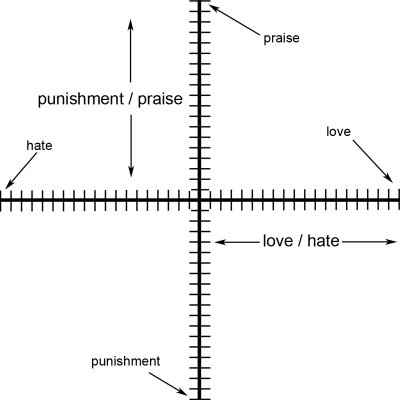Figure 12

The vertical axis is a synonym word-pair of praise and punishment and the horizontal axis is a synonym word-pair of love and hate. It is very important to realize that not only does the words love and hate define the identity of the horizontal or x-axis they also hold conceptual points in space along the axis and the same for praise and punishment with respect to the y-axis. The words which are conceptual points in space define a metaphoric value along its respective axis and can be notated as a coordinate pair similar to (x,y) So you may ask what would a coordinate word-pair look like? Let us look at the two points identified as point 1 or P1(love,praise) and point 2 P2(hate,punishment) (see figure 13.)Figure. 13

Thursday, March 02, 2006

Verbogeometry -- installment II --- Word-Axes and Word-Planes

2. The Mechanics of Verbogeometry:

2.1. Word-Axes and Word-Planes. One of the tenets of Verbogeometry and Mathematical poetry is that you "calculate" values of quality as if they were quantity. Of course this seems to be nonsense but then one must realizes that paradox is the mathematical structure of metaphor. When quality and quantity are synonymous then the math equations automatically transcend the normal duty of denotation and enter into the realms of connotation. This concept also bears some relationship to different concepts of infinity pioneered by the Russian-German Mathematician Georg Cantor, namely the infinities in gradation relative to the infinities in Counting.
Another tenet of verbogeometry is that it recognizes antonyms in only a few varieties. A simple antonym is a word whose antonym is a direct negation. (Example: just / unjust, probable / improbable or fertile / infertile etc.) A complex antonym is a word whose antonym is not a direct negation. (Example: just / unfair, probable / doubtful or fertile / barren etc.) There are also gradable (gradient) antonyms which are pairs that express relationships in a continuum, such as up and down. Complementary antonyms are pairs that express an either/or relationship, such as dead or alive. Verbogeometry uses all antonyms as if they were gradable. It is easy to find examples where poets use complementary antonyms as if they were gradable to create certain metaphors. Example: "Bob showed up half dead to work today."
Within the boundaries of verbogeometry is important to understand that we view words as objects floating in space. When we focus on single words, with no context, they are alone inert relating to no other words. However, when we focus on words that have a synonymous partner we can easily imagine a line in space between the words. Probable and improbable are good examples of simple antonyms that we can view connected by a line. (See figure. 3)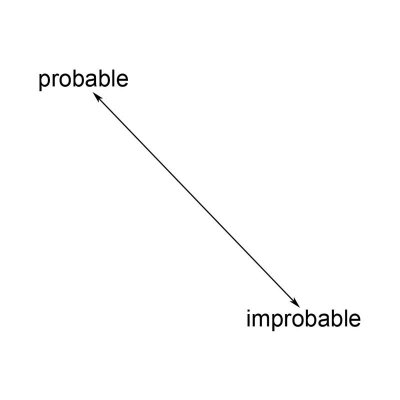Figure 3.

Life is full of dualities it is hard to think about qualities without thinking about opposing ideas. We can view our 'probable / improbable' one-dimensional line as a number line but instead of values of numbers on the line, we think in terms about having different levels of meaning between the two words residing at each end of the line. Due to a number-line being a one dimensional axis it is easy to visualize a word-axis as an axis for a single spatial dimension. We call any pair of words connected by a line a word-axis. Two perpendicular number lines or word axes make a two-dimensional word-axis as well as defining a word-plane. We also have the ability to view the word-plane as an infinite number of coordinates delineated by the word-pairs much like the infinite number of coordinate pairs contained within a Cartesian coordinate system described in the realm of analytic geometry.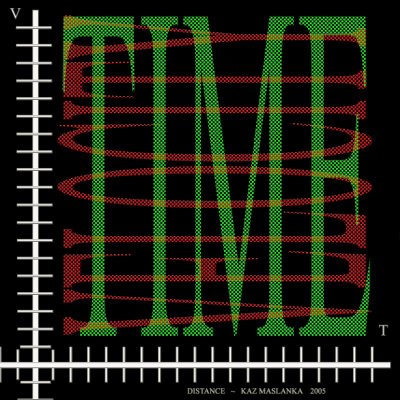Figure 4.

Figure 4 shows a visualization for the physics equation; distance = velocity multiplied by the time. Notice that the y axis displays velocity and the x axis displays time. When we multiply and blend the words in an infinitesimal weave, we arrive at the concept of distance in a tessellated product of the concepts of velocity and time. In another words by positioning the two axes perpendicular to each other, we view every value on one word-axis in relation to every value on the other word-axis. This method affords us a way to 'feel' the entire word plane or axis system with all its different augmented values and gradations. When we multiply two word-axes together we conceptually tessellate a two dimensional plane with different semantic values of the two words blended and augmented. If we were to take a normal Cartesian coordinate system and multiply the x positive integers (1 through 12) times the y positive integers (1 through 12) we get a tessellated plane as in Figure 5 (see figure. 5) (notice the intensity of blue relative to the value of the numbers)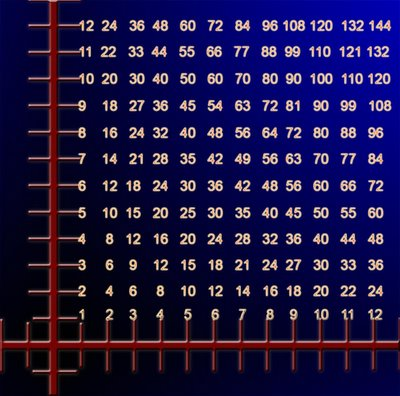Figure 5.

To help us further visualize this concept let us create a word axis using the words red and green. In this instance, we are going to use red and green as nouns instead of adjectives. (We will use colors as adjectives later) Let us multiply a red-green axis times another red-green axis and view it visually. (See figure. 6) Multiplication of colors is similar to color addition except the disparate intensities of the colors are greater and follow a similar pattern shown in figure 5. The value of the 'numbers' is subjective and not as important as the relationship between the 'numbers'.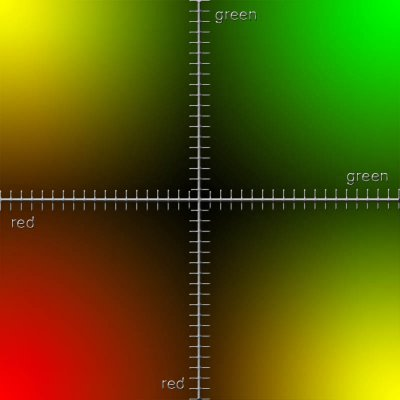Figure 6.

Figure 6 helps us to visualize different word meanings spread across a word plane.
Let us create another example using two different word-axes. However, let's use two different colored word axes instead of both axes being the same colors like our previous example. Then let us break down what we have just accomplished and look at the axes separately. After that we will superimpose a set of different word-axes upon our color axes to compare how the system works.
To facilitate visualizing two different word axes lets look at an example with the word-axes red-green and blue-orange multiplied by each other and mapped on a Cartesian coordinate system. (See figure. 7)Figure 7.

(Note these diagrams are visual aids not scientific data)

Figure 7 is comprised of two axes: a red-green in the vertical and a blue orange in the horizontal. Let us look at the red-green vertical axis separately (See figure. 8)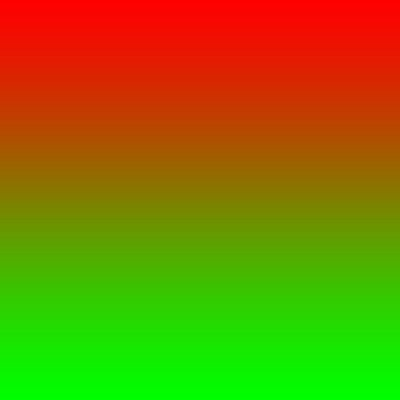Figure 8.

and separate the blue-orange horizontal axis as well (See figure. 9)Figure 9.

Furthermore, notice the direction of the gradations. When we place the axes perpendicular to each other and multiply them together, we see the color tessellations of the word-plane. (See figure. 7)
What is nice about using colors for our examples is that words used for colors function as both a noun and an adjective depending on our intent. When we map a word plane with word-axes that comprise colors and we use them as adjective synonyms, then this word-plane serves as a paradigm or a pedagogical tool to serve as a general model for understanding all two axes synonym word-planes. Example: Let create a word-plane using the two word-axes of noble/ignoble and just/unjust. (See figure. 10)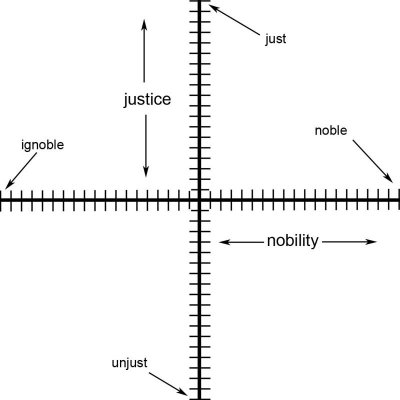Figure 10.

The next step would be to superimpose the noble/ignoble; just/unjust word-plane onto our previous word-plane of blue/orange; red/green. In essence, we are pretending color blue to mean ignoble, orange to mean noble, red to mean just and green to mean unjust. Now we can see the meanings blend into each other in the different areas of our word-plane.(See figure.11)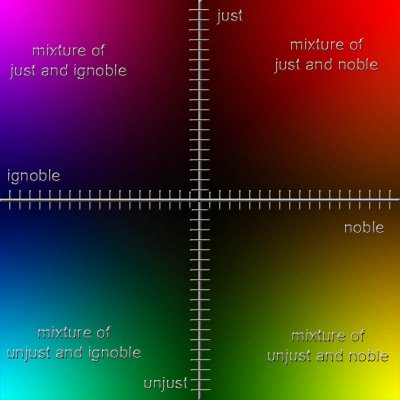Figure 11.

We can see the color purple as blend of ignoble and just, red-orange as a blend of just and noble, yellow-green as a blend of noble and unjust and blue-green as a blend of ignoble and unjust. For the record, I certainly am not trying to say there is a relationship of ignobility and injustice with the color blue-green! This example is just a tool to help us with our own concept of visualizing a word-plane. However, we could create a different but, in my opinion, limited set of color metaphors for noble/ignoble and just/unjust. Or we could look at our color example as adjectives on their own merit. This method would automatically help us see them as metaphors. Example: She was red hot. He had a blue day. He was so green he did not know what was happening. Multiplying adjective word-axes together instantly create metaphors.

Wednesday, March 01, 2006

Verbogeometry -- installment I --- Cartesian Coordinate System

I would like to step through my paper on verbogeometry in small installments if possible. The paper is laid out so that I discuss a mathematical idea and then show how it relates to verbogeometry. The first installment is an overview on how the Cartesian coordinate system works and how it is applied to a remedial physics problem. Next installment will be about the mechanics of verbogeometry.

VerbogeometryThe confluence of words and analytic geometry

1. Cartesian Coordinate System:
Before we can talk about verbogeometry, we must have a look of some pertinent elements in analytic geometry. Concerning this paper on verbogeometry, you should know enough analytic geometry to plot points and a few basic equations on a Cartesian coordinate system. However, I would like to believe that anyone should be able to enjoy some understanding of verbogeometry. If you need a refresher on the Cartesian coordinate system, you may want to look online. Wikipedia has a nice overview of the System.When we look at the two-dimensional axis of a Cartesian coordinate system, we can see that by picking a point somewhere on the plane defined by this axis that there is a relationship of this point back to the origin. This relationship is understood by the nomenclature of the coordinate pair (x,y) where x and y are distances along each axis from the origin. Furthermore, if we draw lines from a newly created point, perpendicular to both the x-axis and the y-axis and taking into consideration the axis system in the background then we will make a rectangle. (See figure. 1)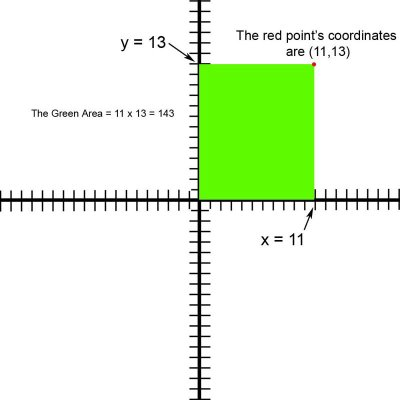Figure1.

The area of a rectangle is product of the lengths of its sides and in the upcoming example the product of the values for the x and y coordinates of this point. Of course this one is a special case because we used the axis origin as our starting point. Example: Let us arbitrarily pick a point defined by the x-y coordinates of (11,13) and draw lines perpendicular to the axes to illuminate what I just said. The area of any rectangle is equal to its height multiplied by its base (The product of the lengths of its sides). We have a green rectangle delineated on our axis system. (See figure. 1) The height of our rectangle is 13 units and it base it 11 units. The area of our rectangle is 143 square units … or 13 X 11 = 143 This later example is one of pure mathematics. However if we want to use math as a language then we will have to apply concepts or words to our axis system. Let us look at a typical physics problem of distance, velocity and time displayed on a two dimensional axis system. Let us assign the y-axis to be levels of velocity in units of miles per hour and the x-axis to be amounts of time in units of hours. (See figure. 2)

The distance of an object traveling in space is equal to the velocity of the object multiplied by the average time it is traveling in space or d=vt. Let us use the same pure mathematical example as before but by our contextualizing the axis and assigning the y-axis to represent velocity and the x-axis to represent time, our original point from the last example (11,13) has a new meaning. To reiterate … the point before was in the realm of pure mathematics but now the point represents a moment in time of a speeding object. The object is traveling 13 miles per hour and has been traveling for 11 hours. So to calculate, (d = vt), the distance the object has traveled we must multiply the velocity by the time or 13 miles per hour times 11 hours which equals 143 miles.In essence, what we have done has been to assign a concept by using words (velocity and time) to our axis system. Moving our attention up or down on the y-axis displays different values of velocity. Moving left and right on, the x-axis displays different amounts of duration or time. Physical experimentation can easily verify the veracity of this equation. In addition, the same experimentation verifies the verbal concepts and their relationship to each other that we have assigned to our axis system. We can see the relationship between the concepts of distance, velocity and time spread out on a two-dimensional plane via our axis system. It is important to note that these concepts occupy orthogonal spaces as well as all equations in the form of A = BC. Furthermore, the equation makes sense again because it matches our perceptions of the event and the axis system is a model of our experience. Although the use of the latter equations was for the purpose of denotation, Verbogeometry is more interested in the use of equations for connotation and witnessing dissimilar concepts forced into orthogonal spaces to create metaphor.

Friday, February 24, 2006

Introducing Verbogeometry

Life has been real busy lately so unfortunately I haven’t made a blog entry for a while. One of the things that have been demanding my time has been my paper on verbogeometry. My plan has been to deliver the paper next summer in London at the Bridges conference on mathematical connections in art music and science. I have been excited about finishing the paper for it’s the most important thing I have written to date on mathematical poetry related matters. I have created a link to an expanded version of the paper on my website. I believe this paper really allows one to understand how words and their meanings can be viewed in space as well as how to approach mathematical poetry in general. Some people don’t know how to approach visualizing mathematical operations on words and their meanings. I believe this paper will give one new tools needed to enjoy mathematical poetry and hopefully inspire new poets. I have hardly scratched the surface of this genre. I imagine many who will fly past me discovering large treasures of aesthetic joy. I feel I am part of a beautiful vista while standing in my tiny garden pitched on a mountain side looking at colorful fields of mathpo expanding out to a horizon of mathematical mountains that I know I will never be able to reach much less enjoy.

Although verbogeometry has existed in my work since 1981 I have never tried to explain the mechanics of how it works. I was hoping people would find their way into it. however, that never really happened proving either I am delusional about its importance coupled with the fact that it is a cumbersome task that delivers far too little aesthetic value for the work required to understand it.

or...

it is a worthwhile endeavor
blending languages
which will induce poetic winds
clearing obscured connections
the spiritual world has
to the physical
bringing a new rationality to art
firmly grounding one end of metaphor
to the infinite.

I am certainly not excluding the former and acknowledging that I may be delusional. However we must also recognize that if we artists were not delusional we would never create anything new which strains the norm. For we would never have the energy required to make art in our aesthetic exile. The avante guard never dies but its endless warriors pay dearly, many sacrificing love and fortunes only to melt into the insignificant gloryless noise quantifying a lonely instant in the spectrum of time.

K

Saturday, January 28, 2006

Sentence Structure in Mathematical Poetry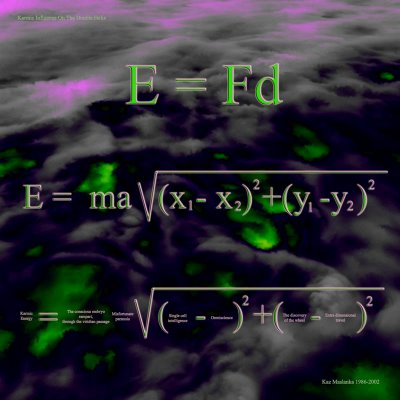Here is a detail of the equation from aboveToday I would like to share part of a conversation I had with Gregory Vincent St. Thomasino dated December 2, 2005 …

Gregory Vincent St. Thomasino said:

You have to find the analogy between math and the grammar of the sentence, and make your "math" work within that grammar.

Kaz Maslanka said:

I am not sure if I understand exactly what you are saying but I find grammatical similarities in physics equations all the time. For example F = ma ---- force is equal to mass times acceleration. “ma” could easily be seen as a clause where mass is the subject and acceleration is the predicate. Many of my polyaesthetic pieces rely on physics equations to supply a sub-context for the piece. Lets look at the piece from “Karmic influences on the double helix” First we must talk a bit about physics. This piece utilizes the physics equation for Energy. E = Fd Energy is equal to force multiplied by distance. In other words the Energy expended on an object is equal to force applied to that object continually spread over a distance. The latter sentence obviously has grammatical qualities and is synonymous with the equation. We also know that F = ma. Now lets break force into its components of mass and acceleration and substitute (ma) for ‘F’ it in our equation that defines energy.

E = (ma)d or E = mad

In other words the Energy expended on an object is equal to the accelerated mass of that object applied continuously over a distance. (Notice again that we are using words to be synonymous with the actual equation)

Even the equation can easily be mapped with sentence structure. The energy in question is equated to Fd or (ma)d where ‘m’ is the subject ‘ad’ is the predicate and d is the object of the verb ‘to accelerate’ To reiterate the mass is the subject and ‘acceleration over a distance’ is the predicate where ‘over the distance’ is the object of the verb ‘accelerate’

Before we look at the poem lets take a look at the distance formula (in two dimensions) used in analytical geometry. In a nutshell the distance formula is the Pythagorean theorem mapped onto a Cartesian coordinate system. e.g. the hypotenuse of a right triangle is the distance we wish to solve and we can do so by using the difference in the y values as one leg of the triangle and the difference in the x values as the other leg of the triangle. This whole mess is a lot easier to see visually. Click here

Now let us look at the poem.

The energy in question is “Karmic Energy” which is equal to the ‘mass’ being the phrases: the conscious embryo, rampart, through the viridian passage” multiplied by the ‘acceleration’ which is “misfortunate paranoia” and multiplied by the distance between the concepts of the difference between the concepts of “the discovery of the wheel and extra-dimensional travel” and the difference between the concepts “single cell intelligence and the discovery of the wheel”

You can see that the poem follows the exact sentence structure of the physics equation, Furthermore the physics equation serves as a paradigm or metacontext for the poem to ‘ride’ or ‘be carried ’ in.

Friday, January 27, 2006

Distance and Visual Poetry

The piece, “DISTANCE”, shows how the concepts of velocity and time tessellate a plane in an infinitely woven tapestry of distance.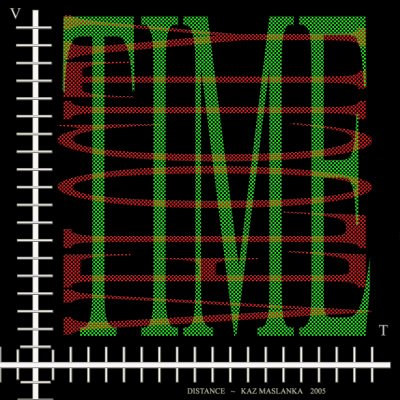The piece above “distance” is a little different for me for it is influenced by new information I have learned from the ‘visual poetry’ movement. The piece has elements of visual poetry that I have never used before. It functions in two different ways. One way it functions mathematically as a visual paradigm for a physics tenant of kinematics. Notice a paradigm is not a metaphor it is a simile. The Second way it functions is mathematical vispo because it performs math operations on text. The bottom line is that I would not call it mathematical poetry as such because we are not performing mathematical operations on words as meaning with the intent for connotation. However we are performing math operations on text and I reiterate that I feel this is in the realm of mathematical vispo. Check out Geof Huths amazing blog if you want to understand all the branches of visual poetry.

On a side note here is a visual poem I made for Gregory Vincent St. Thomasino titled “church” It is inspired by and uses his math poem, “change + purse = church”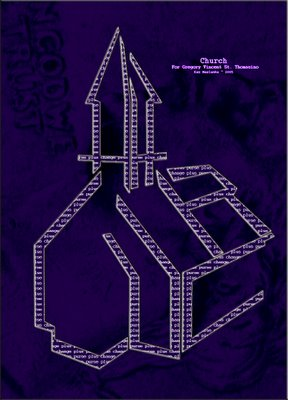Tuesday, January 24, 2006

Four-Dimensionally Centered

This piece was inspired by the beauty in New Mexico and its relationship to the spiritual ideas of its native people. It is a four dimensional point metaphorically pointing at the atonement archetype.
There are four axes in this Cartesian system: the first axis is that of east and west the next axis being north and south the third axis being up and down and the last axis being inward and outward. Mathematically speaking the point described uses the analytic geometric midpoint formula to define the midpoint in each of the four axes. Metaphorically speaking The point described is at the center of everything and nothing.

Wednesday, January 18, 2006

Joint Mathematics Meeting Report

American Mathematical Society

I just returned from the Joint conference on Mathematics in San Antonio and was extremely pleased with the Art Show. Many of the ‘Bridges’ mathartists participated in the show and some attended the conference. Here is a picture of my good friend Reza Sarhangi the nucleus of the Bridges conference (left) standing with Slavik Jablan an extremely important and interesting figure in the Mathart movements in Europe.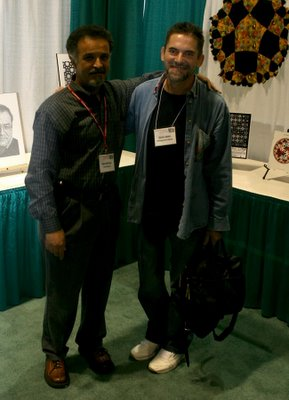Here is a picture of my friend Paul Hildebrandt (left) and Ivars Peterson dining on good Mexican food. Paul is the president of Zometools and Ivars is extremely important figure in the proliferation of fun filled mathematical ideas and is the creator of “Math Trek” for Science News.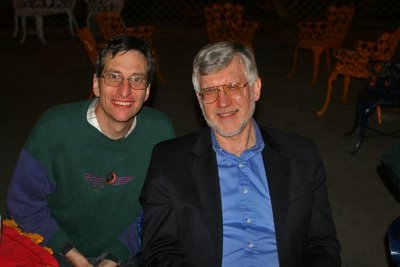Here is a snapshot of Robert Fathauer standing next to his mathart. Robert is president of Tessellations company and he also is involved in the curation of many mathart shows.Here is a snapshot of Francisco Lara-Dammer with his beautiful Cayley diagrams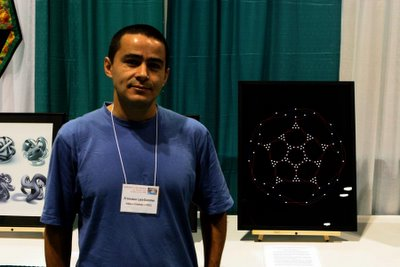Here is a snapshot of Gwen Fisher who does amazing mathematical beadwork.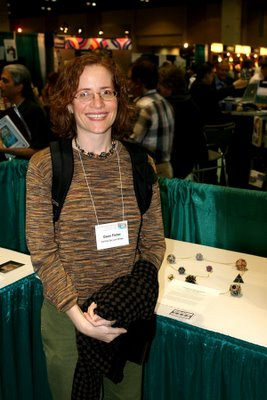Here is a snapshot of Anne Burns and her beautiful mathematical landscapeMy apology to to Bradford Hansen-Smith for missing the opportunity to shoot his picture (he also attended the conference)

Remember the Alamo!Wednesday, January 11, 2006

Mathpo Beauty?

I snagged this quote off of the new-poetry list, submitted by Jeff Newberry

"Life is doubt, and faith without doubt is nothing but death."
Miguel de Unamuno

When I look at this quote I easily see it also as a mathematical metaphor.

Life = Doubt
Faith - Doubt = Death

What hits me so obviously is how much more beautiful the verbal language is relative to its 'seemingly cold' mathematical counterparts. However, the beauty in mathematical poetry is being able to see within an instant all the mathematically synonymous permutations and synergistically experience them as a whole integrated with the poetic beauty and power of the verbal language.

Doubt = Life
Doubt = Faith-death

Life = Faith-death

I wonder if Mr. Unamuno put much thought into the following quote:

Doubt is life, and life is faith without death

Tuesday, January 03, 2006

The Evolution of a Math Poem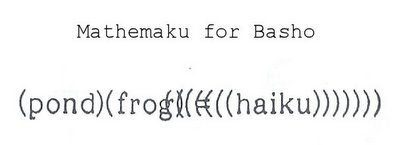Bob Grumman has recently posted another mathpoem worth thinking about. Its history goes back to early March 2005 when Bob was blogging about his version of the Basho haiku which concerns a frog jumping into an ancient pond.

Bobs blog 401

Bobs version:
old pond--abruptly, the sound
of a frog's splash-in

on March 10 2005 Bob reveals that a math poet named El Konde had sent him his interpretation of that same Basho Haiku poem.

Bobs blog 403

Which was:

Old pond / silence = frog splash!

Bob replied, “I like the idea of dividing the pond (arithmetically), but it would be more logical, it seems to me, to divide silence by the frog to get the old pond.” Or:

Silence / frog splash! = Old pond

I would like to analyze these two different syntactical forms of the same Basho poem and see how they relate to Bobs recent poem “Mathemaku for Basho”

First of all we must talk about what takes place when we create and equation in the form:

A = B multiplied by C

When we multiply 2 subjects together those subjects are integrated into a new subject that has its identity is founded in both of the previous subjects.

Lets looks a something we are familiar with If you drive your car 100 miles down the Jersey turnpike from New York to Philly at 50 miles per hour you would get there in about 2 hours (assuming someone doesn’t shoot you for driving too slow)

Distance is equal to velocity multiplied by time. d = v t

The very idea of distance is founded on the ideas of time and velocity at least as far as our equation is concerned. It is also interesting to me that we can use different syntax to make this mathematical expression synonymous in three ways.

d = v t (Distance is equal to velocity multiplied by time.)

v = d/t (velocity is equal to distance divided by time)

t = d/v (time is equal to distance divided by velocity)

Now let’s look at El Konde’s version of the Basho poem:

old pond / silence = frog splash!

Which is mathematically synonymous with:
(silence)(frog splash!) = old pond
and
old pond/frog splash! = silence

What we have done is to integrate the break of silence with the frog splash to define the old pond. Or the (the break of) silence is defined as the old pond divided by frog splash Furthermore we could also read it as the old pond divided by frog splash! Defines (the break of) silence.

I realize that I have added my own interpretation to El Konde’s intention of ‘silence’ in his poem by redefining 'silence' as ‘the break of silence’ but I think it is arguable that Basho was talking about the break in silence as opposed to silence itself. I believe my addition makes El Konde’s version much more clear.

Now let’s look at Bobs change to El Konde’s Poem and its mathematical synonyms:

silence / frog splash! = old pond

silence / old pond = frog splash!

(frog splash!) (old pond) = silence

So what Bob expresses by his change is that the break of silence is defined by the integration of frog splash! and old pond or that the old pond is defined by the break of silence per frog splash and last but not least … frog splash is defined by the break of silence per old pond

Now that we have broke it down, you the reader can make your own decision on what version makes the most sense to you.

Note: (Bob’s intention may have been to think of 'silence' standing as itself, not as 'break in silence', but I doubt it especially since he has ignored it altogether in his new poem)

In conclusion lets look at Bob’s Mathemaku for Basho: http://comprepoetica.com/newblog/blog00691.html

(pond)(frog) = (((((((haiku)))))))

So it shows that Bob is consistent with multiplying pond and frog but he has 'defined haiku' as opposed to defining 'the break in silence'.

By putting parenthesis around the word haiku he has incorporated a vispo technique in his mathematical poem to imply splashing water waves.

So much for evolution,
R Mutt

Wednesday, December 28, 2005

Math Tricks ... Numeric Vispo?

Vê o que acontece se multiplicarmos 37 por múltiplos de 3:

3 x 37 = 111
6 x 37 = 222
9 x 37 = 333
12 x 37 = 444
15 x 37 = 555
18 x 37 = 666
21 x 37 = 777
24 x 37 = 888
27 x 37 = 999

Agora vê isto:

111111111 x 111111111 = 12345678987654321

Repara neste trapézio:

1 x 9 + 2 = 11
12 x 9 + 3 = 111
123 x 9 + 4 = 1111
1234 x 9 + 5 = 11111
12345 x 9 + 6 = 111111
123456 x 9 + 7 = 1111111
1234567 x 9 + 8 = 11111111
12345678 x 9 + 9 = 111111111

E neste outro:

1 x 8 + 1 = 9
12 x 8 + 2 = 98
123 x 8 + 3 = 987
1234 x 8 + 4 = 9876
12345 x 8 + 5 = 98765
123456 x 8 + 6 = 987654
1234567 x 8 + 7 = 9876543
12345678 x 8 + 8 = 98765432
123456789 x 8 + 9 = 987654321

E ainda neste:

0 x 9 + 8 = 8
9 x 9 + 7 = 88
98 x 9 + 6 = 888
987 x 9 + 5 = 8888
9876 x 9 + 4 = 88888
98765 x 9 + 3 = 888888
987654 x 9 + 2 = 8888888
9876543 x 9 + 1 = 88888888
98765432 x 9 + 0 = 888888888
987654321 x 9 - 1 = 8888888888
9876543210 x 9 - 2 = 88888888888

I find these little pieces very interesting.

These have been floating around on the web lately and were emailed to me twice by different people. I have no idea how old they are or who originally discovered them. I would imagine that more than one person contributed to this group. I would not call them mathematical poetry but they may be considered some form of numeric Vispo.

They remind me a little bit of magic squares.
http://mathforum.org/alejandre/magic.square.html

If odd math expressions are your thing you may want to check the following:
http://curiousmath.com/

Happy Holidays

Saturday, December 17, 2005

Golden Fear

GOLDEN FEAR

This aesthetic distillation was inspired by the concept of being told to to fear something that is associated with the golden rule.

The American Mathematical Society has accepted “Golden Fear” in their upcoming show in San Antonio, Texas Here is a link to the other Mathematical Artworks accepted in the show.

http://myweb.cwpost.liu.edu/aburns/jmm06/jmm06.htm

“Golden Fear” uses one of the equations for the golden ratio or phi, which is the most irrational number compared with all irrational numbers. Out of all the equations for phi “Golden Fear” uses the equation, which is in the form of a continued fraction. However, the word ‘fear’ is substituted for the number '1' in the original equationHere is more information on the Golden Ratio.

http://mathworld.wolfram.com/GoldenRatio.html

Monday, December 12, 2005

Power of Confidence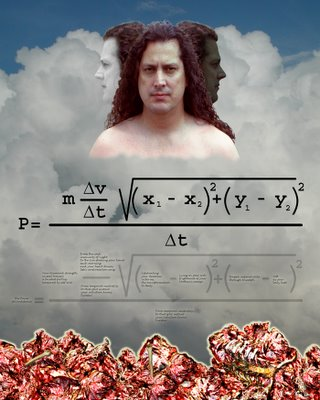Bob Grumman reviewed a couple of pieces of mine on his blog and I would like to share my comments to him.
http://comprepoetica.com/newblog/blog00676.html

Bob,
I appreciate the posting and would like to comment.

Bob said,” To be frank, I'm not sure why he seems to provides a range of values for his delta time,”

Delta means the “change in” see the link below for they explain it quite well. In a nutshell, when delta is present one must put things in terms of what it changes to from what state it originally had. So it goes that the change in x would be between two different states of x. In equation form it follows that (x1-x2) or basically the change from the existing state of x1 to the change of the new state, which is x2. That is why we had two different states in the poem … one being temporal neutrality and the other being the state of eureka

what are deltas?

Concerning the relationship of the Images to the math, I view the relationship of the images to the math poem the same as one would view the music in relation to the lyrics of a song … As to the task of putting my work in terms of Vispo … I guess my work would fit under the category of emblem poems with math poems instead ‘text only’.

Your Blog entry has inspired me to go into detail about how this piece works. The physics equation for Power states that power is equal to energy expended per duration of time. That’s all there is to it … That is the definition of power. (The term work and energy are synonymous)

Understanding the equation for power

However, there is much to what energy means. So now let us look at what physics says about energy.

Different faces of energy

In my piece Energy is the amount of force exerted over a distance. Let’s talk about distance. Distance is basically the Pythagorean Theorem mapped out on a Cartesian coordinate system. What we have is a change in position in one axis relative to a change in position in another perpendicular axis. (The following link explains it quite well)

http://www.purplemath.com/modules/distform.htm

Because there is a relationship between Energy and force lets look at what physics says about force. Force is mass multiplied by acceleration and acceleration is the change in velocity per time. (Delta t) So lets put the puzzle altogether and see how it reads.(in two dimensions) Power is a mass multiplied by a change in velocity per time multiplied by a change in position in one axis relative to a change in position in another axis and all of the previous verbiage is divided by a change between two positions in time.

Now let’s put the equation for power in context with my piece “The power of confidence”

Let me introduce a term … “virtual object” can be an emotional or psychological object present in my mathematical poetry that utilizes physics equations as a paradigm “to carry” the poem. The virtual object is a substitute for the physical object that is normally the subject of a physics equation.

The mass is of our virtual object is “Your diamond strength crystal bravery, a faceted fortress tempered by your self love”

The change in velocity 'delta v' of our virtual object = “From the cold insecurity of night to the sun shaking your hand each morning while your heart drums life's confirmation song”
The change in time that affects the virtual object 'delta t' = “From temporal neutrality to that split instant your intuition knows . . . eureka!”

The distance our virtual object will traverse:
The difference between x1 and x2 in our first dimension:

x1 = “Celebrating your demonic victories, the transformation to deity”

Furthermore, the difference between y1 and y2 in our second dimension:

y1 = “Forged impenetrable through triumph”
y2 = “Soft as your baby butt”

The Poem in words:
So you can see that our mass of “Your diamond strength crystal bravery, a faceted fortress tempered by your self love” is being accelerated between the concepts of “the cold insecurity of night” to the concept of “the sun shaking your hand each morning while your heart drums life's confirmation song” and this is happening between the moments of “temporal neutrality” to the moment of “that split instant your intuition knows . . . eureka!” and all of this is moving over the distance between the difference of the concepts of “Celebrating your demonic victories, the transformation to deity” and “Lying in your crib, frightened of your mothers sneeze” and moving in a second dimension over the distance between the difference of the concepts of “Forged impenetrable through triumph” and “Soft as your baby butt” Furthermore all of this is occurring between the moments of “temporal neutrality” to the moment of “ that split instant your intuition knows . . . eureka!”

Thursday, December 08, 2005

Beginner's Mind ~ Denotation / Connotation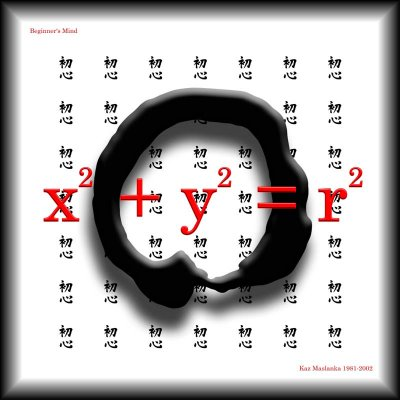There are numerous meanings throughout Asian philosophical history for the term “Beginners Mind”. That said, I will repeat one of my favorite stories illuminating the term in support of this blog post.

In the mathematical visual poem entitled “beginners mind” two forms of perfection are expressed. The first one is the Buddhist calligraphic circle called Enso in practiced in Japan or Ilwonsang practiced in Korea. The painting of this circle is created over and over as the monks practice the meditation of staying in the present moment. While in the present moment the “emptiness of self” can be experienced, thus substantiating this image as a symbol of emptiness.  To us it is a perfect example of circular perfection expressed as connotation … and layered on top of that, we have a perfect example of circular perfection expressed as denotation in none other than the analytic geometrical equation for a circle:

x^2 + y^2 = r^2

While using this equation to plot points creating a circle on a two-dimensional Cartesian coordinate system one becomes aware of the awe-inspiring infinite precision of not only this equation but by conceptual extension into all mathematical equations.

The 49 Chinese characters in the background are the calligraphic Chinese expression of “Beginner’s mind”

Link to the equation of a circle: (my example h and k are valued at zero) http://www.analyzemath.com/CircleEq/Tutorials.html
A moment of silence for John Lennon ...

Monday, December 05, 2005

AMS American Mathematical Society Conference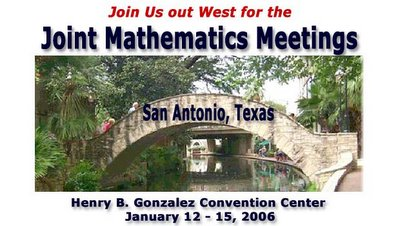I plan on attending this conference ... It should be a good time.

check out their web site.

http://www.ams.org/amsmtgs/2095_exhibits.html

Sunday, December 04, 2005

Mathematical Simile Mathematical Metaphor

Mathematical simile is the function of virtually all applied math equations for which the public is familiar (usually scientific). For example physics equations are paradigms used to correlate experience. Mathematical models can be made for anything rationally understood and are descriptive of the relationships between the elements described.
A simile is a paradigm. One can see this expressed in the physics equation distance is equal to velocity multiplied by time. When you apply this equation to a situation you realize that this is an approximation even for all practical purposes it accomplishes the task at hand. The bottom line is that it is an approximation even though the equation expresses it as exact. The math equation is exact the application is not. Therefore it is simile ‘our experience of distance is like velocity multiplied by time.’ One may argue that the physics equation is metaphor precisely for the same reason e.g. we are saying that distance is exactly equal to velocity multiplied by time and it is not. Therefore we have a logical tension that creates the metaphor. The next step is to look at the intention of the equation, whether it is intended for connotation or denotation. Clearly physics equations are intended for denotation. Therefore what is really important for us is the intention of the equation. This also illuminates the idea that there are a lot of shades of gray between simile and metaphor yet we don’t usually think in this manner. I know a lot of people that think simile and metaphor are really the same. I believe at some point there is a nonsense threshold that one crosses and you can take it no other way except metaphor. Unfortunately that threshold line can be anywhere on a number line between the concept of simile and metaphor. Personally I see that threshold very close to simile. That is as soon as it starts getting nonsensical I consider it metaphor.

The visual image presented takes the following simile and expresses it as metaphor.

Although it may or may not be universally true I find that a man’s intelligence is inversely proportional to the levels of alcohol and testosterone in his system. I gave a lecture last week to a group of art students from Santa Ana Art Institute (Thank you Professor Ben Miles) and when I proposed this statement I got a bunch of laughs and a lot of nodding heads. Consequentially I believe there is some truth in there.Friday, December 02, 2005

Humor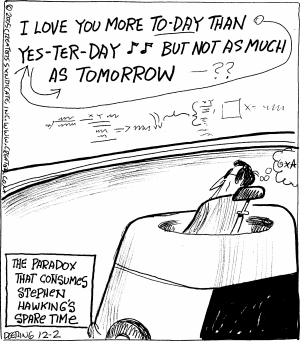Tim Repp sent me this little mathpo related humor

Thursday, December 01, 2005

Synecdochical Physics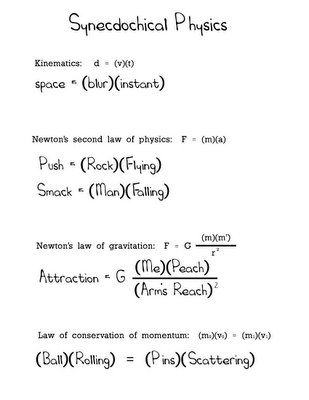I was inspired by conversations with The Mathematician Paul Gailiunas and a blog entry of Visual Poet Geof Huth to produce some synecdoche physics equations.

syn·ec·do·che n. Listen:
A figure of speech in which a part is used for the whole (as hand for sailor), the whole for a part (as the law for police officer), the specific for the general (as cutthroat for assassin), the general for the specific (as thief for pickpocket), or the material for the thing made from it (as steel for sword).

Whereas in all of the equations the variables, for the physics equations, have been replaced with a synecdoche counter part not all of the equations as a whole could be considered synecdochical. All of them but one created a synecdochical equation.

Newton’s equation on gravity is actually a metaphor. That is due to the fact that the force variable (replaced by attraction) in the mathpoem does not pertain to gravity but is in reference to the attraction caused by hunger. If it were pertaining to gravity then the equation would be synecdochical however anything else makes the equation a metaphor.

http://dbqp.blogspot.com/2005/10/synechdoche-explained.html

Friday, November 25, 2005

Math Poets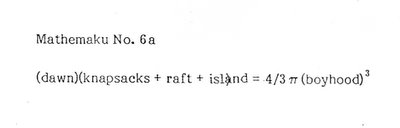I have been delinquent in making entries into the blog but finally I have put some links into the link section. Besides myself I have added a link to a couple of Bob Grumman's pages and one to a Page of Scott Helms. Of all of Bobs Work my favorite is the one above. Bob and I seem to be the only people in the English speaking world who continue to focus on Mathematical Poetry or Math-Po If there are others please let me know. There are others who have done work in Visual Poetry and call their work Mathematical Poetry Karl Kempton is one who has done some very interesting Visual Poetry Using Mathematical operations on text. I would guess that the authority on how Math has been used in Visual Poetry would be Bob Grumman or Possibly Geof Huth. Geof is my focal point on the world of Visual Poetry. The depth of information Geof covers is astounding.
While I am on the subject of Visual Poetry I would like to mention I really like the selected poems by Michael P. Garofalo

Saturday, March 12, 2005

What is mathematical poetry

Mathematical poetry is an artistic expression created by performing mathematical operations on words or images as if they were numbers. One may find this baffling because it seems we are being confused about the states of quality versus quantity. But it is through the fusion of this dichotomy that mathematical metaphor is spawned. Historically speaking we believe that math has never been intended for metaphor. We intend to change that. By blending the wondrous aesthetics of mathematics with enigmatic aesthetics of art we intend to bridge the gap between the infinite and the concrete.